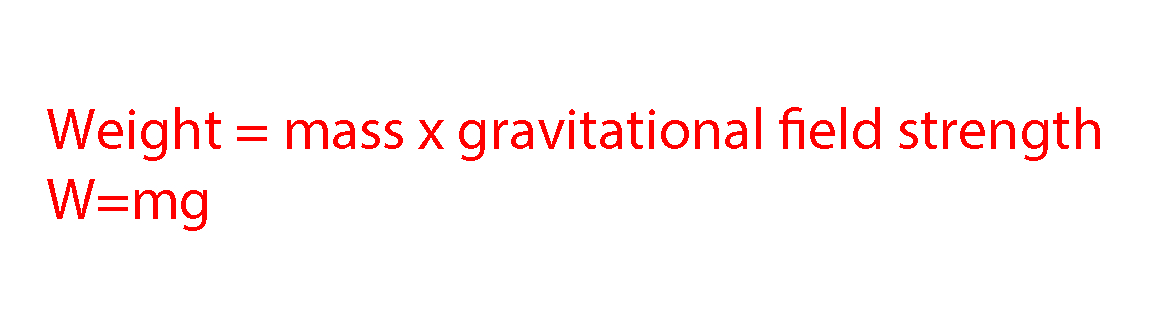# Weight

Weight is the result of gravity on an object's mass. The formula used to calculate weight is:This may also be written as Weight = mass x acceleration due to gravityWeight of a massive gun (weight = mg) held by a newt (units in Newtons).

NOTE: Weight is a force measured in Newtons (N). The weight of an object will vary with the gravitational force that acts upon it. This is why objects weigh less on the moon than they do on earth. The gravitational field strength of the moon is less than the earth's, so when the object is on the moon the force it experiences pulling it down (its weight) is less than it would be on earth.

Example 1

The surface gravity of Mars is 3.7N/kg. On Earth it is 9.8N/kg. Beagle 2, the unsuccessful British space probe, had a mass of 30kg. What was the weight of Beagle 2 on Earth before launch? And how much less did it weigh on the surface of Mars?

Weight = mass x gravitational field strength                                     W = mg

Weight on Earth = 30 x 9.8 = 294N

Weight on Mars = 30 x 3.7 = 111N

Difference in weight = 294 – 111 = 183N less on Mars

Example 2

In December 1995, NASA’s Galileo probe crashed into Jupiter’s atmosphere. If its weight on Earth was 3.4kN and Jupiter’s gravity is approximately 23N/kg, whilst on Earth it is roughly 10N/kg, what is the Galileo probe's mass? And what was its weight as it crashed into Jupiter?